Next: Example 7.3: Kirchhoff's rules Up: Electric Current Previous: Example 7.1: Ohm's law

## Example 7.2: Equivalent resistance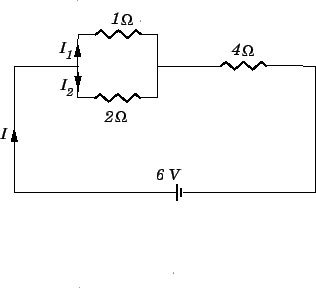Question: A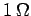and a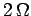resistor are connected in parallel, and this pair of resistors is connected in series with a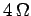resistor. What is the equivalent resistance of the whole combination? What is the current flowing through theresistor if the whole combination is connected across the terminals of a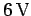battery (of negligible internal resistance)? Likewise, what are the currents flowing through theandresistors?

Answer: The equivalent resistance of theandresistors is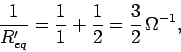giving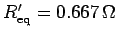. When a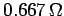resistor is combined in series with aresistor, the equivalent resistance is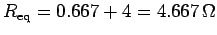.

The current driven by thebattery is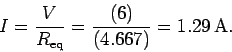This is the current flowing through theresistor, since one end of this resistor is connected directly to the battery, with no intermediate junction points.

The voltage drop across theresistor is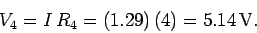Thus, the voltage drop across theandcombination is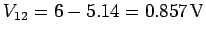. The current flowing through theresistor is given by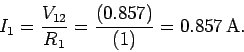Likewise, the current flowing through theresistor is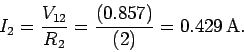Note that the total current flowing through theandcombination is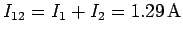, which is the same as the current flowing through theresistor. This makes sense because theandcombination is connected in series with theresistor.Next: Example 7.3: Kirchhoff's rules Up: Electric Current Previous: Example 7.1: Ohm's law
Richard Fitzpatrick 2007-07-14